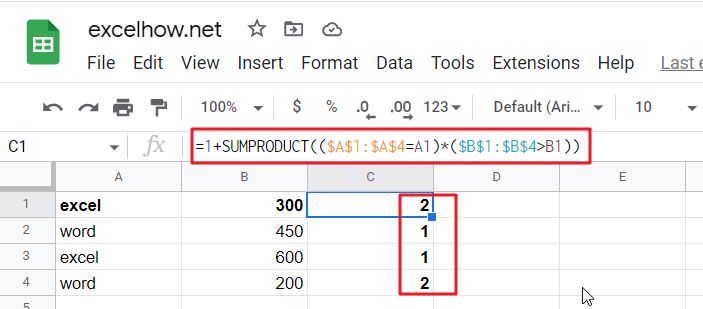## Excel/Google Sheets: Full Row Reference

Excel or Google sheets supports both full row references and full column references. This article will talk about the usage of full row references.

Full Row reference is another cell reference in Excel or Google Sheets, which is used to reference the entire Row, for example, if you want to reference the entire Row 1, you can use the following reference format 1:1.

## Full Row references

A full Row reference is composed of two Row numbers plus a colon, the starting cell and the end of the cell is joined together by a colon. In the full- Row cell references, there is no Column letter, if you want to identify the entire Row of cells, you only need to use two Row numbers on it. For example, you can use 1:1 to indicate Row 1, you can use 1:2 to indicate Row 1 and Row 2.

When you want to sum all the cells in Row 1, then you can use the SUM function in combination with the full Row reference 1:1, the formula is as follows.

`=SUM(1:1)`

Full Row references are suitable for a large number of columns or dynamically changing the number of columns in the data table, it can be easily used and entered through the method of full row references.

Full row references have a big disadvantage is the performance problem, when the row contains a large number of cells or invalid cells, it will lead to very low performance.

## SAMPLE Files

Below are sample files in Microsoft Excel and Google sheets that you can download for reference if you wish.

Google Sheets Sample file: click here.

## Excel/Google Sheets: Full Column Reference

Full column reference is another cell reference in Excel or Google Sheets, which is used to reference the entire column, for example, if you want to reference the entire column A, you can use the following reference format A: A.

## Full column references

Full column reference is composed of two column numbers plus a colon, the starting cell and the end of the cell is joined together by a colon. In the full-column cell references, there is no row number, if you want to identify the entire column of cells, you only need to use two column numbers on it. For example, you can use A:A to indicate column A, you can use A:B to indicate column A and column B.

When you want to sum all the cells in column A, then you can use the SUM function in combination with the full column reference A: A, the formula is as follows.

`=SUM(A:A)`

Full column references are suitable for a large number of rows or dynamically changing the number of rows in the data table, it can be easily used and entered through the method of full column references.

Full column references have a big disadvantage is the performance problem, when the column contains a large number of cells or invalid cells, it will lead to very low performance.

## SAMPLE Files

Below are sample files in Microsoft Excel and Google sheets that you can download for reference if you wish.

Google Sheets Sample file: click here.

An expanding reference is a reference type in Excel and Google worksheets that extends the range of a cell when a formula is copied to the cell below or to the right. In this article, we will explain how to use extended references in Microsoft Excel or Google worksheets with specific examples.

## Expanding reference

Extended reference is mainly achieved through a mixture of reference methods, including absolute and relative references. When you want to position a cell region, you can set the first cell as an absolute reference, set the last cell as a relative reference, so that you can constitute an extending reference, for example: \$A\$1:A1.

## EXAMPLE 1

When you want to add up data from the same column, you can use Expanding reference to do it in Excel or google sheets. In this example, the data that needs to be added up is the cell range A1:A6, and the following steps are used to achieve the summation by expanding the reference.

Step1: Enter the following summation formula in cell B1 and press Enter to apply the formula.

`=SUM(\$A\$1:A1)`

Where, \$A\$1:A1 is the Expanding Reference cell range.

Step2: In cell B1, drag the AutoFill Handle until cell B6

Step3: You can click on any cell in the B1:B6 area to see its summation formula.

## EXAMPLE 2

Suppose you have a list of names and you want to mark the number of occurrences of each name. For example, the first occurrence of the name TOM is marked as 1, the second occurrence is marked as 2, and so on. This can be easily achieved by using the COUNTIF function in combination with the Expanding Reference method.

The formula is as follows:

`=COUNTIF(\$A\$1:\$A1,A1)`

## SAMPLE Files

Below are sample files in Microsoft Excel and Google sheets that you can download for reference if you wish.

Google Sheets Sample file: click here.

In Microsoft Excel Spreadsheet or Google sheets, there is another cell reference, mixed references, where part of the reference is absolute, part of the relative. This article will describe how to use mixed references through specific examples.

## Mixed Reference

When the formula is copied to other cells, it is only used to maintain the absolute position of one of the rows or columns of the referenced cell in Excel or Google Sheets, while the direction of the other positions are changed, this type of reference is called mixed references.

Mixed references can be categorized into absolute column and relative row(=\$A1) and relative column and absolute row(=A\$1) references.

For example: formula: =\$B1, when the formula is copied to the right, it will always keep \$B1 unchanged, and the row number will change when copied down, that is, row relative and column absolute references.

## EXAMPLE

If you have a table in which the B1:J1 cell range entered the number 1-9, A2:A10 cell range also entered 1-9, we want to calculate the intersection of B1: J1 and A2: A10 two cell range of cell multipliers, this time, you have to use a mixed reference. You can enter the following mixed reference formula in cell B2.

`=\$A2*B\$1`

If you want to be able to fix a cell address when copying a formula, then simply use the absolute reference symbol \$ before the row or column number.

## Quickly toggle between reference types

The shortcut keys F4 or Fn+F4 are provided in both Excel and Google Sheets to toggle between different reference types. For the formula = A1, press F4 or Fn+F4 to switch the order of the shortcut keys as follows.

`\$A\$1->A\$1->\$A1->A1`

## SAMPLE FILE

Below are sample files in Microsoft Excel and Google sheets that you can download for reference if you wish.

In Microsoft Excel spreadsheet or Google Sheets, the cell reference is the cell or cell area address or name in the worksheet, the cell reference contains two types of references, namely, relative references and absolute references.

## Absolute Reference

An absolute reference in Excel or Google Sheets means that when copying a formula to another cell in a worksheet, the absolute position or absolute reference of the cell referenced in the formula will never change.

Absolute reference is a cell reference by adding the dollar (\$) sign in front of the column name and row number so that the row and column remain unchanged.

For example, in cell B2, enter the absolute reference type formula: = \$A\$1, then whether the formula is copied down or to the right, it will always remain as = \$A\$1.

## EXAMPLE

Suppose you have a product price table A1:B6, you need to calculate the discount price of each product whose discount value is in cell E1, that is, you need to multiply the price value of each product by the discount value in cell E1, for this case, you need to use absolute references, the formula is as follows：

`=B2*\$E\$1`

The detailed steps are as follows.

Step1: We enter the following absolute reference formula in cell C2, and then press the Enter key

`=B2-B2*\$E\$1`

Step2： In cell C2, drag the Fill Handle until cell C6.

Step3: You can click on any cell in C2:C6 to see its absolute reference to the formula, you can see that the reference position of cell E1 has not changed.

## Quickly toggle between different reference types

Although the use of different cell reference methods can be set as needed for convenience, but manually enter the “\$” symbol or more tedious.

There are shortcut keys F4 or Fn+F4 provided in Excel and Google Sheets, which can be toggled between different reference types. For the formula = A1, press F4 or Fn+F4 shortcut key to switch the order as follows.

`\$A\$1->A\$1->\$A1->A1`

This is much faster and easier than entering the \$ character manually.

## SAMPLE FILE

Below are sample files in Microsoft Excel that you can download for reference if you wish.

## Conditional formatting based on another column in Google Sheets or Excel

In Microsoft Excel Spreadsheet or google sheets, conditional formatting is used to highlight any cell based on a predefined condition and the value of those cells. In the previous article, we described how to format a specific column or cell range based on another cell value in Excel or google sheets.

In Excel or Google sheets, it is relatively easy to apply conditional formatting based on the current value of a cell. However, suppose you run into a problem where you have to highlight or format a column based on the value of another column. You can use conditional formatting based on another column to solve such problems.

This article will describe in detail how to format another column based on another column’s value in a Microsoft Excel spreadsheet or Google Sheets.

## EXAMPLE

The following is an example that can be used to demonstrate the application of conditional formatting functionality in an instance. Suppose you have a product sales table with a sales target and an actual sales value for each month, now let’s use conditional formatting to find the product names whose actual sales are higher than the sales target. Below is a screenshot of our product sales table.

Below we will introduce how to customize conditional formatting rules by formulas to format cells in one column based on the value of another column in Microsoft Excel Spreadsheet and Google sheets respectively.

## Highlight Cells Based on Another Cell in Excel

If you want to highlight a column based on the value of another column, then you can create custom conditional rules in Conditional Formatting. The specific steps are as follows.

Step1: Select the column to which you want to apply the custom condition rule, for example: A3:A7.

If you want to apply conditional formatting, you must first select the cells. If you want to highlight the entire row after applying conditional formatting, you need to select all the data sets. But to highlight the cells of a column, you only need to select the cells of that column. If you want to highlight an entire row or column, then you need to select that column or row.

In the example in this article, you need to select the name column first, i.e. A3:A7

Step2: You need to open the Conditional Formatting panel and select the appropriate formatting rule as needed. In Ribbon, select HOME->Conditional Formatting -> New Rule, New Formatting Rule dialog will open.

Note: To apply conditional formatting to a column based on another column, we need to select the New Rule menu.

Step3:  From the list of rule types below, we can see the type of rule we need, that is, we want to determine the cells to be formatted by a formula. We need to select: Use a formula to determine which cells to format.

Note: As you can see from the image above, the New Formatting Rule dialog box has a number of rule types, such as: Format all cells based on their values, Format only cells that contain, Format only top or bottom ranked values, Format only values that are above or below average , Format only unique or duplicate values and Use a formula to determine which cells to format.

When the formatting formula returns a True value, the cell will be applied to the corresponding format, and if the formula returns False, then the formula will not be applied.

Step4: When you select the “Use a formula to determine which cells to format” rule type, the box named “Format values where this formula is true ” will appear below the “Select a Rule Type” box. Enter the following formula in the Format values where this formula is true text box:

`=\$C3>\$B3`

Note: The formula above compares the value of column C with the value of column B in the same row. If the value of column C is greater than the value of column B in the same row, then the cells in column A will be formatted.

Step5: Click the “Format” button to select a format for the cells that match the criteria. After setting your preferred formatting style, click the OK button.

Step6: You will see your selected formatting style in the Preview box of the New Formatting Rule window. After clicking OK, the selected formatting will be applied to the cells that meet the conditions.

## Highlight Cells Based on Another Column in Google Sheets

The following is a conditional formatting function to highlight the cells in the name column that match the condition based on the column value in the Google Sheet spreadsheet. The steps are as follows.
Step1: In this case, select the product name column (excluding the title) A3:A7 and it will be highlighted in blue.

Step2: Click the “Format” menu and then click the “Conditional Formatting” menu.

Or right-click on the selected column -> select “View more cell actions” -> “ Conditional formatting “, the Conditional formatting rules dialog box will pop up.

Step3: Next we can select the desired format for the range or column. Select Single color Tab

Note: We can also select the range of cells by manually selecting them in the Conditional Formatting Rules window. For example, if we choose to conditionally format the first 100 cells of column A, we can specify the range to apply to as “A3: A103”.

Step4: Select “Custom formula is” from the “Format Cells if” drop-down list

Step5: In the Formula text box, enter the following formula.

`=\$C3>\$B3`

The formula here is the actual sales value corresponding to the product name cell is greater than the sales target value. The formula becomes “=\$C3>\$B3” because the actual sales value is in column C with the first cell row number of 3, and the sales target value is in column B with the first cell row number of 3.

The formula uses the greater than operator (>) to evaluate each cell in C3:C7 to the corresponding cell in B3:B7. When the formula returns “true”, the rule is triggered and the highlighting format is applied.

Step6: Select a style from the “Formatting style” drop-down list

Step7: Click the “Done” button

As you can see from the image above, you will see that the cell containing the name will be conditionally highlighted based on the value of another column.

## Conditional Formatting Based On Another Column FAQ

How do you conditional formatting in Excel based on another column text?

1. Select the cell to which you want to apply conditional formatting. Click the first cell in the range, then drag it to the last cell.
2. Click Home > Conditional Formatting > Highlight Cell Rules > Included Text…
3. Select the color formatting for the text, and then click OK.

How do you conditional format if one column is greater than another?

1. Do one of the following to open the dialog box. choose Data > Conditional Formatting > Highlight Cell > Greater Than.

Can you use conditional formatting to compare two columns?

1. If you want to compare two columns and highlight the matching data, you can use the duplicate function in conditional formatting.

How do you conditional format a range based on another range?

1. Select all cells in the worksheet that you want to highlight and apply formatting rules to.
2. Click Conditional Formatting.
3. Select the Highlight Cells rule, and then select the rule that applies to your needs…
4. Fill out the Less Than dialog box and select a formatting style from the drop-down menu.

## Conclusion

This article provides you with a base method for conditional formatting of an entire column based on another column in Excel and Google sheets. Therefore, if you follow the steps described in this article, you will be able to apply conditional formatting to columns based on the conditions of other columns.

## Sample files

Below are sample files in Microsoft Excel and google sheets that you can download for reference if you wish.

## Conditional Formatting based on Another Cell in Google Sheets/Excel

In Microsoft Excel Spreadsheet or google sheets, when you want to format a specified cell or cell range based on the value of a different cell, for example, formatting the first row based on the value of a cell in a single column, you can use the conditional formatting function to create formatting formulas.

This article will talk in detail about how to format a cell range based on the value of another cell in a Microsoft Excel spreadsheet or Google Sheets.

## EXAMPLE

The following is an example that can be used to demonstrate how to apply conditional formatting functions in an instance. Suppose you have a table of student scores and you need to determine the names of students with scores greater than 85. Below is a screenshot of our sample student score table.

There are several ways to find and print out the names of students with grades greater than 85 in Excel or Google Sheet. You can use the IF function to do a score comparison and then print out the cell values that meet the criteria. Another way is to use the conditional formatting function to quickly highlight the names that meet the criteria.

## Conditional format function introduction

You can easily highlight specific columns with the conditional formatting function, because the cells to be formatted are highlighted if they are the same as the cells to be evaluated or if they match a condition. That is, we can format the cell state of the column in which the same type of value is located based on the value of a cell as a condition.

To accomplish the above and simply highlight the status of the Score column, the following steps can be performed.

STEP 1: Select the Score column

STEP2: Click HOME Tab, in the Styles group, click Conditional Formatting command, click Highlight Cells Rules menu, and then click the cell rules in the secondary menu, such as: Greater Than, Less Than, Between, Equal To ,etc.

In this example, we select the Equal To cell rule. Then the Equal To dialog box will pop up.

Step3: We can enter 75 in the Format cells that are EQUAL TO: text box, select the corresponding highlighted state in the with drop-down list, and then click “OK“.

Step4: Excel will apply the selected format to the specific cell that meets the condition.

The method described above clearly does not meet our goal of highlighting the state of a specific column or other columns based on the value of a specific cell. That is, we need to format a specific cell or cell range in the Name column based on the value of cell E2.

When the built-in conditional rules of Excel or Google Sheets do not meet our goal, i.e., formatting other different cells based on a specific cell, then we need to use Excel or Google Sheets formulas to customize the formatting rules to meet our goal.

Below we will introduce how Microsoft Excel spreadsheet and Google Sheets respectively can customize conditional formatting rules via formulas to format cells in the Name column based on the value of cell E2.

## Highlight Cells Based on Another Cell in Excel

If you want to highlight a cell range based on the value of another cell, then you can create custom conditional rules in Conditional Formatting. The specific steps are as follows.

Step1: Select the cell range where you want to apply the custom conditional rule, such as A2:B7

Step2: In the Ribbon area, select HOME->Conditional Formatting -> New Rule, the New Formatting Rule window will open.

Step3:  From below the list of rule types, we can see the type of rule we need, and that is we want to determine which cells to format by a formula. We need to select: Use a formula to determine which cells to format.

Note: As you can see from the image above, the New Formatting Rule dialog box has a number of rule types, such as, Format all cells based on their values, Format only cells that contain, Format only top or bottom ranked values, Format only values that are above or below average , Format only unique or duplicate values and Use a formula to determine which cells to format.

When the formatting formula is set to return a True value, the cell will apply the corresponding formatting, if the formula returns False, the formula will not be applied.

Step4: Enter the following formula in the Format values where this formula is true text box

`=\$B3>\$E\$2`

Note: As you can see from the formula above, cell E2 needs to use absolute references to limit cell changes, you need to enter the \$ sign in front of the row and column values.

Step5: Click the “Format” button to select a format for the cells that meet the conditions.

Step6: When you click OK, the selected format is applied to the cells that meet the conditions.

## Highlight Cells Based on Another Cell in Google Sheets

The following is a conditional formatting function in the Google Sheet spreadsheet to highlight the cells in the name column that match the condition based on the cell’s value. The steps are as follows.

Step1: Select the cell range A3:A7 in the name column

Step2: Click on the Format menu, and then click on the Conditional formatting menu

Step3: The Conditional formatting rules window will open.

Step4: Select Single color Tab

Step5: Select “Custom formula is” from the “Format Cells if” drop-down list

Step6: In the Formula text box, enter the following formula.

`=\$B3>\$E\$2`

Step7: Select a style from the “Formatting style” drop-down list

Step8: Click on the “Done” button

As you can see from the image above, you will see that the cell containing the name will be highlighted based on the score condition in the adjacent cell.

Note: When our custom conditional formula returns True, the cells that meet the condition will be formatted, when it returns False, google sheets will not do anything.

## Multiple conditional rules

If you want cells that meet more than one condition to apply formatting, then you can use the logical functions AND and OR, for example, you want to highlight cells or ranges with a score greater than 90 and the name carol. Then you can use the following formula to customize the conditional rules.

`=AND(\$B3>\$E\$2,\$A3="carol")`

The effect after applying the format is as follows.

## Conditional Formatting Based On Another Cell FAQ

How do you conditionally format a cell based on another cell?

1. Select the cell you want to format…

2. On the Home tab, in the Styles group, click Conditional Formatting > New Rule…

3. In the New Formatting Rule window, select Use Formulas to Determine Which Cells Need to be Formatted.

4. Enter the formula in the appropriate box.

5. Click the “Format… ” button to select your custom format.

Can I use an IF formula in conditional formatting?

The answer is both yes and no. Any conditional formatting parameter must produce a “true” or “false” result, i.e., your conditional formatting rule is an If/Then statement that says “If this condition is “true”, format the cell in this way”.

Can you use conditional formatting based on relative cell references?

This cell reference type is rarely used in Excel conditional formatting rules.

Can you drag conditional formatting?

Yes, Select the cell and apply the conditional formatting, referencing other cells in the row. Drag the corner of the row down to the bottom of the cells you want to apply the formatting

## Conclusion

Once you learn the conditional formatting function in Excel or Google Sheets, you will easily format other cells or ranges based on specific cell values, which is a is a very useful method.

## SAMPLE FILES

Below are sample files in Microsoft Excel and google sheets that you can download for reference if you wish.

## Compare Two Strings in Excel/Google Sheets

This article will talk about how to compare two given strings in a Microsoft Excel spreadsheet or Google Sheets. How to compare two strings in Excel by using VBA macros to see if they are the same, if they are the same then return TRUE, if not then return FALSE.

Suppose there is a worksheet with two columns A,B containing text values, if you want to compare the text values of two peers in columns A,B to see if they are the same, how do you do the comparison in Excel or Google Sheets? Below we will compare text values by formula and VBA code.

## Method1: Compare Strings Through Excel/Google Sheets Formula

In Microsoft Excel Spreadsheet or Google sheets, you can use the EXACT formula to compare two strings. When two text values are the same, the EXACT function will return TRUE, otherwise it will return FALSE.

### Generic Formula

`=EXCEC(TextValue1,TextValue2)`

For the example in this article, we can use the following EXACT formula:

`=EXACT(A2:B2)`

You need to enter the above EXACT formula in cell C3, after that you can drag the auto-fill handle from cell C3 to cell C7 so that the other cells can also apply the above EXACT formula.

### Explanation

EXACT functions are case-sensitive by default, for example.” Excel” and “EXCEL” are two different text values. If you want to compare two text strings in a case-insensitive manner, then you can simply use the “=” operator.

The formula is as follows:

`=A3=B3`

For case-sensitive cases, we can use the EXACT function to compare two strings.

Since EXACT returns TRUE or FALSE results by default, if you want to have a more friendly output, then you can embed the EXACT function into the IF function so you can define different output results.

The formula is as follows.

`=IF(EXACT(A3,B3),"equal","not equal")`

## Method2: Compare Strings Through VBA Macro

When you want to compare strings, if you want to compare strings to get the result of which string is smaller or larger than the other, which is impossible to achieve through the EXACT function, then how to solve this problem? This problem can be perfectly solved by the StrComp() method in Excel VBA, which can compare two strings and get the desired value of the result.

• If the two strings you want to compare are equal, this method will return 0.
• If the first string is smaller than the other, you will get -1 as the return value.
• If the first input string is greater than the other string, you will get the value 1 as the result.
• If one of the strings entered by the user is null, the return value will be Null.
• If you want to compare “excel” and “Excel”, then you can execute the following VBA code:
``````Sub comparetest()

Dim compare As Integer

string1 = "Excel"

string2 = "excel"

comparestring = StrComp(string1, string2)

MsgBox comparestring

End Sub``````

## Compare effect of (non-annual) compounding periods on growth

This article will talk about how to calculate the trend or effect of future value changes for different compounding periods in a Microsoft Excel spreadsheet or Google Sheets via a formula.

## What is the period of compound interest?

The compounding period is the time interval at which the bank calculates the interest rate on unpaid cash and usually corresponds to the payment period, but not necessarily so.

The payment period is the time interval over which payments are made. The size of the periodic payments on a given loan is related to the interest rate and the length of the loan term. The longer it takes the borrower to pay off the loan, the more interest he/she will pay to the bank. In addition, the more often a borrower makes annual payments to the bank to reduce the outstanding balance (e.g., monthly rather than annually), the less interest he/she will have to pay over the entire repayment period. When the borrower and lender agree on the loan amount, interest rate and loan term, it is easy to calculate the amount to be repaid in each installment.

## What is FV?

Future value (FV), also known as future value, is the value of a certain amount of money at some point in the future. It is usually recorded as FV.

Example: The ultimate value of simple interest formula.

• FV: Future value;
• PV: Present value;
• Rate: Interest rate (discount rate);
• Periods: The number of periods for calculating interest

## Calculation of the future value

The formula for calculating the future value of simple interest is as follows:

` FV = PV * (1 + n * i)`

The formula for compound interest future value is as follows:

`FV=PV * (1 + i)n`

## General Formulas in Excel / Google Sheets

You can use the following general formula in Excel or Google Sheets to calculate the future value of an investment based on a constant interest rate, as follows

`=FV(rate,nper,pmt,[pv],[type])`

The syntax of the FV function has the following parameters.

•  Rate – This is a mandatory parameter, which is the interest rate per period.
• Nper – This is a mandatory parameter, the total number of annuity payment periods.
• Pmt – This is a mandatory parameter, the amount of each payment; it cannot be changed during the lifetime of the annuity. Normally, pmt includes the principal and interest, but not other fees or taxes. If pmt is omitted, the pv parameter must be included.
• Pv  – This is an optional parameter, final or future value. If pv is omitted, it is assumed to be 0 (zero) and you must include the pmt parameter.
• Type – This is an optional parameter with a number of 0 or 1, indicating the time of payment. If type is omitted, it is assumed to be 0.

## Example

For the worksheet shown above, we can use the FV function to calculate the future values for different compounding periods so that we can clearly see the impact of compounding periods on growth. Through the data table, we can see that the initial value is 5000, its annual investment rate is 7.5%, the Periods column is the different compounding periods, and then we can calculate the future value PV of 5000 by using the FV function, you can enter the following formula in cell C3 of the Excel or google worksheet.

`=FV(F3/B3,B3*F4,0,-F2)`

## Formula explanation

Let’s explain how the above general formula works. The FV formula is used in Microsoft Excel or Google Sheets to calculate compound interest and return the future value of an investment over a specified period. To use this formula, then you need to provide some necessary parameters, such as: interest rate, number of periods, present value, etc.

For the above example.

Present value is cell F3.

The interest rate is determined by dividing the annual interest rate value by the number of periods, i.e. F3/B3

The number of compounding periods is the value of the terms multiplied by the number of periods, i.e. F4*B3

With these parameter values above, we can calculate the future value of the different periods.

## Q&A

1. How do I calculate compound interest in Excel with different rates？

A more efficient way to calculate compound interest in Excel is to apply the general interest formula: FV=PV(1+r)n, where FV is the future value, PV is the present value, r is the interest rate per period, and n is the number of periods of compound interest.

2. What will be the effect if the compounding period is increased?

Increasing the number of compounding periods increases the effective annual interest rate compared to the nominal rate.

3. Which is better compounded monthly or annually?

Due to compounding, the annual interest rate is usually a higher rate. Instead of monthly payments, the amount invested has a 12-month increase. However, if you can get the same interest rate for monthly payments as for annual payments, then take it.

## What is the Coefficient of Variation?

The coefficient of variation is the ratio of the standard deviation of the original data to the mean of the original data. The coefficient of variation has no scale, so an objective comparison can be made. In fact, the coefficient of variation can be thought of as an absolute value that reflects the degree of dispersion of the data, just like the extreme deviation, standard deviation and variance. Its data size is influenced not only by the degree of dispersion of the variable values, but also by the size of the mean of the variable values.

## Calculation of coefficient of variation

The coefficient of variation is calculated as:

`C.V = (standard deviation / mean ) × 100%`

If the coefficient of variation is small, the degree of variation (deviation) is small and the risk is small; conversely, if the coefficient of variation is large, the degree of variation (deviation) is large and the risk is large.

Note: The magnitude of the coefficient of variation is influenced by the mean and standard deviation statistics, so it is better to list both the mean and standard deviation when using the coefficient of variation to express the degree of variation of information.

## General Formula

You can use the following general formula in Excel or Google Sheets to calculate the coefficient of variation, as follows.

`=STEDV.P(A1:A5)/AVERAGE(A1:A5)`

where A1:A5 is the sample data to be calculated and the data needs to be in the same row.

## Formula Explanation

Let’s explain how the above general formula works. In order to calculate the coefficient of variation in Excel or google sheets, you need to use the STDEV.P function in a formula combined with the AVERAGE function. In the example in this article, you need to enter the following formula in cell H3.

`=STDEV.P(A3:E3)/AVERAGE(A3:E3)`

You need to calculate the standard deviation using the STDEV.P formula by entering the following formula in cell G3.

`=STDEV.P(A3:E3)`

Then drag the Fill Handler to cell G4 and apply the formula above. You will see that the difference between the standard deviation values in G3 and G4 is very small. So in order to distinguish the degree of their variation, you need to calculate the coefficient of variation, which can be done by entering the following formula in cell H3.

`=G3/AVERAGE(A3:E3)`

This formula will first calculate the mean of the A3:E3 range and then divide the mean by the standard deviation value in G3 so that the coefficient of variation can be calculated. You will then need to drag the fill handler from cell H3 to cell H4 in order to apply the above formula.

From the values in H3,H4 you can see that the difference is still significant.

## Q & A

### 1. What is CV instatistics Excel?

The coefficient of variation, also known as the “standard deviation rate,” is another statistical indicator that measures the degree of variation in the observations in a data set. When comparing the degree of variation of two or more data, if the units of measurement are the same as the mean, the standard deviation can be used directly for comparison. If the units and/or the mean are different, the degree of variation cannot be compared using the standard deviation, but rather the ratio of the standard deviation to the mean (relative value).

The ratio of the standard deviation to the mean is called the coefficient of variation or C.V. The coefficient of variation eliminates the effect of different units and/or means on the comparison of the degree of variation of two or more data.

### 2. How do we calculate the coefficient of variation?

The formula for calculating the coefficient of variation is: Coefficient of variation = (standard deviation/mean) × 100%.

### 3. Why do we need to calculate the coefficient of variation?

When you need to compare two sets of data, if the measurement scales of the two sets of data are too different, or there are differences in the scales of the data, it is not appropriate to compare them directly with the standard deviation, then you have to eliminate the influence of the measurement scale, and the coefficient of variation can do this, it is the ratio of the standard deviation of the original data to the mean of the original data.

### 4. What is the difference between coefficient of variation and standard deviation?

Both standard deviation and coefficient of variation are common statistical indicators used to describe the dispersion of data.

Standard deviation: It is an absolute indicator of the dispersion of each observation centered on the arithmetic mean. The standard deviation cannot be used to compare the dispersion of two sets of data when the overall mean is different or when the units of measurement are different.

Coefficient of variation: The ratio of the standard deviation to the mean is called the coefficient of variation, denoted as C.V. The coefficient of variation eliminates the effect of different units and/or means on the comparison of the degree of variation of two or more data.

### 5. What is the coefficient of variation and do you have an example?

Which of the two breeds of adult sows has a greater degree of variation in weight, when the average weight of adult sows in a breeding farm is 200 kg with a standard deviation of 10 kg, while the average weight of adult sows in category B is 206 kg with a standard deviation of 8 kg.

Although the observed values in this case are all the same weight per unit, their means are not the same and the coefficient of variation can only be used to compare their degrees of variation.

Coefficient of variation for the weight of adult sows of breed A: CV = 10/200 x 100% = 5%

Coefficient of variation for the weight of adult sows of breed B: CV = 8/206 x 100% = 3.88%.

Therefore, the degree of variation in body weight of adult sows of breed A is greater than that of adult sows of breed B.

### 6. If the variance is 15 and the mean is 70, what is the coefficient of variation?

The coefficient of variation is: 21%. (15/70=0.21)

## Phone Number Format in Excel

Sometimes when we import phone numbers from external sources, the formatting of the phone numbers is removed. Even if in some situations the phone numbers are not imported from external resource, sometimes the phone numbers in worksheet are not displayed in the correct format, which is very common in daily work. When we are faced with a string of unformatted phone numbers, we find it very confusing and difficult to see the layout of the phone number. Therefore, it is very important for us to learn how to format phone numbers in Excel worksheets and Google worksheets.

In today’s tutorial, we will introduce how to format phone numbers through Excel built-in cell format function, Excel function or formula. These functions can be applied directly to Microsoft Excel worksheet and Google sheet to format phone numbers. We can also customize the formatting in excel worksheets and google worksheets to format different phone number formats depend on different countries.

In addition to how to format phone numbers, we also introduce the phone number formats of some countries, including USA, UK, France, China, Brazil, etc. Each country offers two phone number formats depending on the international or domestic call you are dialing from. We also list the ways to format phone numbers for these countries.

## Format Phone Numbers to Built-in Excel Format

Let’s start with the built-in “Format Cells” function in Excel to convert number format to phone number format.

In excel, there is a self-contained phone number format. This feature is available in Excel worksheets, Google worksheets. In worksheet, there is a special format in “Format Cells” options to format phone numbers according to the position of the numbers. The layout of phone numbers may vary from country to country in the number of digits and symbols, such as the placement of parentheses and hyphens. Here are the specific steps to perform this operation.

Below steps are operated on Excel 2016 for Mac.

STEP 1# Select the cell or a list that needs to be formatted.

STEP 2# In the Home tab (Home tab is displayed on the top in the ribbon), select the Format drop-down menu, then select “Format cells…“. You can also just right-click on your selection and select “Format Cells…” from the pop-up menu.

STEP 3# In the pop-up “Format Cells” dialog box, select the fist “Number” tab, click “Special” from the Category list, then you can see a list of formatting types on the right side of the dialog box.

STEP 4# Select the “Phone Number” option from the list. Then you can see that your phone number is formatted correctly in Sample field. In our example, 2134567890 is formatted to (213)456-7890.

STEP 5# Click “OK” to apply this format to all phone numbers in the selected range.

It should be noted that the format of the cell can not be text, this function does not work well for text. If there is a leading “0”, we need to remove this “0” first, and then use custom formatting to add “0” back. Let’s look at the following example of custom formatting.

## Format Phone Numbers to A Custom Format

If you do not find a preset format that meets your requirements in Excel worksheet or Google worksheet, you can set up a custom format via “Format Cell” function to display the numbers as phone numbers. Again, we still use the “Cell Format” setting to apply custom format to the numbers. Here are the steps for formatting a phone number using custom format.

STEP 1# Select the cell or a list that needs to be formatted.

STEP 2# In the Home tab (Home tab is displayed on the top in the ribbon), select the Format drop-down menu, then select “Format cells…“. You can also just right-click on your selection and select “Format Cells…” from the pop-up menu.

STEP 3# In the pop-up “Format Cells” dialog box, select the fist “Number” tab, click “Custom” from the Category list, then you can see a list of formatting types on the right side of the dialog box. Some types are preset; some are saved in daily work per your demand.

STEP 4# You can select a built-in format that is most similar to the one you want to create. If there is not a similar one, you can type a proper phone number format you want in “Type” textbox. Then custom phone number format is reflected in Sample field. Note that all symbols entered, including spaces, will be reflected in the phone number format.

Here we enter (###) ###-#### to reflect phone number. Each # represents a number.

STEP 5# Click “OK” to apply this format to all phone numbers in the selected range.

Custom format helps us format phone numbers without restrictions. Using a good concatenation symbol, we can enter any phone number format we want. We can also enter zero and plus sign directly into the custome phone number format.

## Phone Numbers with Preceding “+” Sign or Leading “0”

Enter (0###) ###-#### in Type:

Enter 0 (###) ###-#### in Type:

You can add +1 in front of all phone numbers:

Enter (+1) (###) ###-#### in Type:

Using the types listed above we can add a leading 0 or country code (or area code) to a phone number if the original phone number does not have a leading 0 or code.

## Format Phone Number with Excel TEXT Function

The text function can quickly handle phone numbers with the same format.

TEXT function syntax:

`=TEXT(value, format_text)`

### Format a phone number

In the cell just next to phone number, enter =TEXT(A1,”(###) ###-####”).

### Format a phone number table

In my example, the phone numbers are saved in a table, so when you enter the TEXT function in B2, the value parameter is the phone numbers table, and when you press ENTER to run TEXT, a new table is created.

### Format phone numbers with different formats (different lengths)

If phone numbers of different lengths are mixed together, the TEXT function alone cannot help us format the phone numbers, so we need the help of the IF function. We can first sort the phone numbers by region or length of the phone number, and then use the IF and TEXT functions to format the phone numbers. Note that this formula can only format two types of phone numbers.

For example, the length of the phone number is different. Some contain 11 digits and some contain 10 digits. For phone number with 11 digits, format text to “(###) # ###-####”, for phone number with 10 digits, format text to “####-###-###”.

`=TEXT([@[Phone Number]],IF(LEN([@[Phone Number]])<11,"####-###-###","(###) # ###-####"))`

## List of International Phone Number Format Standards

International standards for phone number formats are issued by the International Telecommunication Union. Each country and region has its own way of writing phone numbers. Even for the same phone number, the format of the phone number is different for domestic calls and international calls.

A standard phone number should be “+ (country code) (area code) phone number”. For example, +(1)(425) 555-0100 is the phone number for the U.S. If you are calling a U.S. phone number, their phone number starts with +1, followed by the correct 10 digits of the phone number (the first three are the area code). The other countries follow the similar rules.

When dialing an international call, you need to add 001 (the U.S. international call area code) plus the U.S. local area code in front of phone number or cell phone number.

For example, in the dialing screen, type 001 860 xxxx xxxx, you can call Connecticut from abroad. Here 00 is the international call access code, the leading 0 is usually ignored in writing.

US Phone Number Format

International Call +1 860 XXX XXXX

Domestic Call +0860 XXX XXXX

(The prefix code 860 is the area code for Connecticut, USA)

UK Phone Number Format

International Call +44 20 XXXX XXXX

Domestic Call +020 XXXX XXXX

(The prefix code 20 is the area code for London, UK)

Australia Phone Number Format

International Call +61 2 XXXX XXXX

Domestic Call +02 XXXX XXXX

(The prefix code 2 is the area code for Sydney, Australia)

People’s Republic of China Phone Number Format

International Call +86 10 XXXX XXXX

Domestic Call +010 XXXX XXXX

(The prefix code 10 is the area code for Beijing, RPC)

Japan Phone Number Format

International Call +81 3 XXXX XXXX

Domestic Call +03 XXXX XXXX

(The prefix code 3 is the area code for Tokyo, Japan)

South Korea Phone Number Format

International Call +82 2 XXX XXXX

Domestic Call +02 XXX XXXX

(The prefix code 2 is the area code for Seoul, South Korea)

India Phone Number Format

International Call +91 80 XXXX XXXX

Domestic Call +080 XXXX XXXX

(The prefix code 80 is the area code for Bangalore, India)

### Related Functions

• Excel IF function
The Excel IF function perform a logical test to return one value if the condition is TRUE and return another value if the condition is FALSE. The IF function is a build-in function in Microsoft Excel and it is categorized as a Logical Function.The syntax of the IF function is as below:= IF (condition, [true_value], [false_value])….
• Excel Text function
The Excel TEXT function converts a numeric value into text string with a specified format. The TEXT function is a build-in function in Microsoft Excel and it is categorized as a Text Function. The syntax of the TEXT function is as below: = TEXT (value, Format code)…

## Check Cell If Contains One of Many with Exclusions

In Microsoft Excel Spreadsheet or google sheets, when cells contain multiple strings, how can we accomplish this task if you want to check whether these cells contain more than one given string and exclude other given strings?

In this article, we will discuss how to check if a cell contains more than one given string and exclude other given strings by using a formula. You can create a new nested formula by using the SEARCH function (google sheets or Excel), the ISNUMBER function and the SUMPRODUCT function.

The generic formulas are as follows.

`= ( SUMPRODUCT ( -- ISNUMBER (SEARCH(INCLUDE_STRINGS, TEXT_STRING))) > 0 ) * ( SUMPRODUCT ( -- ISNUMBER (SEARCH(EXCLUDE_STRINGS, TEXT_STRING))) = 0 )`

## Check Cell If Contains One of Many with Exclusions in Google Sheets & Excel

Suppose you have two data tables A3:B12, E1:F4, you want to check whether the cell area A3:A12 contains one of the values in the cell range E2:E4, but does not contain any of the values in the cell range F2:F3. You can build the following google sheets or Excel formulas based on the above general formula.

`=(SUMPRODUCT(--ISNUMBER(SEARCH(INCLUDE_STRINGS,A4)))>0) * (SUMPRODUCT(--ISNUMBER(SEARCH(EXCLUDE_STRINGS,A4)))=0)`

where INCLUDE_STRINGS is the named range E2:E4, and EXCLUDE_STRINGS is the named range F2:F3.

You can enter the above formula in cell B4 and press Enter to apply the formula.

Let’s see how this formula works：

= ( SUMPRODUCT ( — ISNUMBER (SEARCH(INCLUDE_STRINGS, A4))) > 0 )

This formula is used to check whether cell A4 contains INCLUDE_STRINGS range of strings, SEARCH function will find from A4 contains INCLUDE_STRINGS range of strings, if it exists, will return the position of the string in cell A4, otherwise return # VALUE!

ISNUMBER formula will SEARCH function return value into TRUE or FALSE, when the search function returns a number, ISNUMBER function will return TRUE, otherwise it will return FALSE.

The double negative sign before ISNUMBER converts the TRUE or FALSE value returned by the ISNUMBER function to 1 and 0.

=(SUMPRODUCT(–ISNUMBER(SEARCH(EXCLUDE_STRINGS,A4)))=0)

This formula is used to check whether the cell does not contain any of the values in F2:F3. Where the SEARCH function is used to perform a lookup string and return the position of the string in cell A4 or return the #VALUE! error message.

## Related Functions

•  Excel ISNUMBER function
The Excel ISNUMBER function returns TRUE if the value in a cell is a numeric value, otherwise it will return FALSE.The syntax of the ISNUMBER function is as below:= ISNUMBER (value)…
• Excel SEARCH function
The Excel SEARCH function returns the number of the starting location of a substring in a text string.The syntax of the SEARCH function is as below:= SEARCH  (find_text, within_text,[start_num])…
The Google Sheets SUMPRODUCT function multiplies corresponding components in the given one or more arrays or ranges, and returns the sum of those products.The syntax of the SUMPRODUCT function is as below:= SUMPRODUCT (array1,[array2],…)…

## If Cell Contain Specific Text

This article will explain how to check if a cell contains text or a specific text string in Google Sheets or Microsoft Excel spreadsheets. In our daily work, we often look for specific text in worksheets containing large amounts of data or need to determine if a specific cell area contains text values.

We may also encounter the need to find out if a cell contains partial text or we need to find a specific text string in a cell by using VBA code.

## Cell Contains Specific Text in Excel & Google Sheets

Below we will show how to check if a cell contains a specific text by using a formula in Excel or Google Sheets.

If you want to check if a given cell contains a specific text, then you can use the SEARCH function in combination with the ISNUMBER function to create a nested formula. You can refer to this general formula below.

`=ISNUMBER(SEARCH(specific_text,text))`

In the general formula above, specific_text is the specific text you want to find, and text is the text string or cell reference you want to find that contains the specific text.

If you found a specific text, then the formula will return TRUE, otherwise it will return FALSE.

If you need to find partial text from the cell, then the SEARCH function is very suitable; by default, the SEARCH function will automatically find partial text to match.

For the example in this article, you can use the following formula to find specific text.

`=ISNUMBER(SEARCH(B4,A4))`

From the screenshot above, we can see that the formula supports partial text matching, where specific_text is “rest” and the text is “interest dividends“, and the result returned is TRUE, which means that partial text matching for a specific character is successful.

Let’s see how this formula works:

When the SEARCH function finds a specific text, it returns the position of that text in the searched text cell, and if not found, it returns #VALUE! . So, we can take advantage of this feature by simply using the ISNUMBER function to check if the SEARCH function returns a number, and if it is a number, it means that the specific text was found in the cell.

For the ISNUMBER function, it returns TRUE if the given argument is a number, otherwise it returns FALSE. therefore, when the SEARCH function can return the position of a specific character, then the ISNUMBER function returns TRUE. if the SEARCH function returns a #VALUE error, then the ISNUMBER function will return FALSE.

## Cell Contains Specific Text with Case Sensitive in Excel & Google Sheets

You can use the SEARCH function to find specific text and partial text matches, but the function is not case-sensitive.

If you want to check for specific text in a case-sensitive manner in a text cell, then you cannot use the SEARCH function, and you need to replace the SEARCH function with the FIND function, using the following formula.

`=ISNUMBER(FIND(B4,A4))`

As you can see from the above figure, specific_text “Rest” can no longer be successfully matched in text cell A4.

## If Cell Contains Specific Text Then in Excel & Google Sheets

If you want to do something else when you find a cell contains a specific text, for example, we do not want to return TRUE or FALSE results, but rather return some more meaningful text or results.

You can build an IF nested formula by nesting the above formula into the IF function as follows.

`=IF(ISNUMBER(SEARCH(B4,A4)),"Found", "No Found")`

Note: Because this formula uses the SEARCH formula, the result is case-insensitive.

## Sum If Cell Contains Text in Google sheets & Excel

If a column of cells contains a specific text string, you want to add the corresponding cell value of another column, then you can use the SUMIFS function contains a specific text of the cells corresponding to the data of another column to sum. The general formula is as follows.

=SUMIFS(sum_range, criteria_range,specific_text)

If you want to find all the cells in the cell region A4:A12 contains the “expen” string, and the corresponding value of another column B4:B12 to sum, then you can use the following formula.

`=SUMIFS(B4:B12,A4:A12, "*expen*")`

If you are using google sheets, then you can also accomplish this task by using the following formula, which is as follows.

`=SUM(filter(B4:B12, regexmatch(A4:A12,"expen")))`

As you can see from the chart above, the formula consisting of the SUM function and the FILTER function returns the same result as the EXCEL formula.

Note: Excel does not have a special wildcard formula that uses the “*” meta-character to match any character. There is a special regular expression function regexmatch in google sheet.

## Conditional Formatting If Cell Contains in Google Sheets & Excel

In Microsoft Excel, if you want to highlight a text cell containing the “expense” string, then you can refer to the following steps.

STEP 1: Select the cell range A4:A12, then in the HOME tab, click the Conditional Formatting button, and then click the New Rule submenu.

STEP2: The New Formatting Rule window will pop up, click on “Use a formula to determine which cells to format“, then enter the following formula in the text box.

`=SEARCH(“expense”,A4)`

STEP3: Click the Format button and select a color to fill the cells that can be matched successfully. Click OK.

STEP 4: You will see that all cells that contain the expense string are automatically highlighted.

In google sheets, if you want to highlight a text cell that contains an “expense” string, then you can refer to the following steps.

STEP1: Select the cell range that contains a specific text to be highlighted by conditional formatting

STEP 2: Click Format menu, then click Conditional Formatting submenu, the “Conditional format rules” window will pop up on the right side of the page.

STEP 3: Select Text Contains in the Format rules drop-down list and enter the specific text string “expense” in the text box

STEP 4: Select a highlight color in Formatting style and click the Done button.

STEP 5: Let’s see the final result.

## Related Functions

•  Excel ISNUMBER function
The Excel ISNUMBER function returns TRUE if the value in a cell is a numeric value, otherwise it will return FALSE.The syntax of the ISNUMBER function is as below:= ISNUMBER (value)…
• Excel SUM function
The Excel SUM function will adds all numbers in a range of cells and returns the sum of these values. You can add individual values, cell references or ranges in excel.The syntax of the SUM function is as below:= SUM(number1,[number2],…)…
• Excel SEARCH function
The Excel SEARCH function returns the number of the starting location of a substring in a text string.The syntax of the SEARCH function is as below:= SEARCH  (find_text, within_text,[start_num])…
• Excel Find function
The Excel FIND function returns the position of the first text string (substring) from the first character of the second text string.The FIND function is a build-in function in Microsoft Excel and it is categorized as a Text Function.The syntax of the FIND function is as below:= FIND  (find_text, within_text,[start_num])…
• Excel SUMIFS Function
The Excel SUMIFS function sum the numbers in the range of cells that meet a single or multiple criteria that you specify. The syntax of the SUMIFS function is as below:=SUMIFS (sum_range, criteria_range1, criteria1, [criteria_range2, criteria2], …)…
• Excel Filter function
The FILTER function extracts matched records from a collection of data using one or more logical checks. The include argument specifies logical tests, which might encompass a wide variety of formula conditions.==FILTER(array,include,[if empty])…

## Cell Contains Number

This article will talk about how to check if a given cell contains any numbers in Microsoft Excel Spreadsheet or Google Sheets. How to quickly check if a cell contains a number? When you have a small number of cells that need to filter out the cells that contain numbers, you can manually select them, and that is acceptable; however, when there are thousands of cells, it is not wise to filter the cells with numbers by manually.

Below we will see how to quickly check whether the cell contains numbers by Excel or google sheets formula, when the cell contains numbers, return TRUE, otherwise return FALSE.

## Cell Contains Number in Google Sheets & Excel

In Excel or google sheets, if a cell contains letters and numbers, then we can assume that it is a text cell.

### Generic Formula

If you need to test whether a given text cell contains any of the numbers, then you can use the FIND function in combination with the COUNT function consisting of nested formulas. The general formula is as follows.

`=COUNT(FIND({0,1,2,3,4,5,6,7,8,9},A3))>0`

where A3 is the text cell reference you want to test, the formula will go to check whether the text cell A3 contains the given number, and the number to be checked in the form of an array nested into the FIND formula.

### Formula Explanation

#### = FIND({0,1,2,3,4,5,6,7,8,9},A3))

First, we need to use the FIND function in the given text cell to find out the location of the corresponding number in the text, if the number is found, then it will return the location of the number in the text string, if not found, then return # VALUE!

For example: the formula FIND (“2”, A3), the number 2 is in the position of 7 in cell A3, then the FIND function will return 7. Just see the below screenshot.

Since we pass the FIND function an array containing numbers, the FIND function will return a similar array as the return value.

```{8,9,7,10,#VALUE!,#VALUE!,#VALUE!,#VALUE!,#VALUE!,#VALUE!}
```

Note: You can also replace the FIND function with the SEARCH function to find the position of a number in a text string, so the SEARCH function can return the same result.

#### =COUNT(FIND({0,1,2,3,4,5,6,7,8,9},A3))

The array result returned by the FIND formula is passed to the COUNT function as an argument, and the COUNT function counts the items in the array. Since the COUNT function only counts numeric values, all # VALUE! items in the array will be considered as 0.

When there are no numeric items in the array, the COUNT formula will return 0, otherwise it will return a value greater than 0.

For example: we use COUNT formula to count the array values {1,3,#VALUE!}, it returns 2, see the screenshot below:

#### =COUNT(FIND({0,1,2,3,4,5,6,7,8,9},A3))>0

When we count the number of numbers contained in the text cell, then we need to compare the number of numbers is greater than 0, if greater than 0, then return TRUE, if equal to 0, then return FALSE.

We just need to compare the value returned by the COUNT formula with 0, see the below example:

`=COUNT({1,2,#VALUE!})>0`

As you can see from the screenshot above, the COUNT function returns 2, which is greater than 0, so it returns TRUE.

## IF Nested Formulas

If you want to make the return value look more meaningful, then you can embed the COUNT formula above into the IF function so that it returns YES or No, see the following formula.

`=IF(COUNT(FIND({0,1,2,3,4,5,6,7,8,9},A3))>0,"yes","NO")`

## Check Cell Contains Number in Google Sheets

You can use the generic formula above in Google Sheets to check if a text cell contains a number.

Note: Now you can pass array values directly in Google sheet, so you don’t need to use the CTRL+SHIFT+ENTER shortcut to turn the formula into an array formula. Of course, you can use the shortcut to convert it to an array formula and the result is the same.

`=ARRAYFORMULA(COUNT(FIND({0,1,2,3,4,5,6,7,8,9},A3))>0)`

See the below screenshot:

You can see that the returned value is the same as the formula above.

## Related Functions

• Excel IF function
The Excel IF function perform a logical test to return one value if the condition is TRUE and return another value if the condition is FALSE. The IF function is a build-in function in Microsoft Excel and it is categorized as a Logical Function.The syntax of the IF function is as below:= IF (condition, [true_value], [false_value])….
• Excel FIND function
The Excel FIND function returns the position of the first text string (sub string) within another text string.The syntax of the FIND function is as below:= FIND(find_text, within_text,[start_num])…
• Excel COUNT function
The Excel COUNT function counts the number of cells that contain numbers, and counts numbers within the list of arguments. It returns a numeric value that indicate the number of cells that contain numbers in a range…

## Cash Denomination Calculator

Every country has different cash denominations, so you may need to calculate the number of different denominations based on the total amount. If it is just a small amount of cash, then you can calculate the different cash denominations manually, but if multiple cash amounts are needed to calculate the number of denominations, then it is not a good way to do it by manually.

This article will explain how to quickly calculate a given cash denomination value using formulas in a Microsoft Excel spreadsheet or Google Sheets.

## General Formulas for Money Denomination Calculators

In Excel or Google Sheets, you can calculate the cash denomination number by using the following INT function and in combination with the SUMPRODUCT function to create a nested formula as shown below.

`=INT((\$A4-SUMPRODUCT(\$B\$3:B\$3,\$B4:B4))/C\$3)`

For the denomination values in the first column, the following formula needs to be used.

Note: When you execute this formula, you need to make sure that iterative calculation is turned on, otherwise you will see the error message #VALUE!

#### To turn on the Iterative calculation, do the following：

For Microsoft Excel, you need to go to the Excel options window, then go to the Formulas category, and in the Calculation options area, check the box “Enable iterative calculation“. See the following screenshot.

For Google sheets, you need to select the File menu, then click on the Settings submenu, the “Settings for this Spreadsheet” window will pop up, click on the Calculation tab, set the Iterative calculation status to On, and then click on “saving settings“.

### Let’s See How To Use This Formula

STEP 1: Enter the following formula in cell B4 and press Enter to make the formula take effect.

`=INT(\$A4/\$B\$3)`

STEP 2: Select cell B4, drag Fill Handler down to the other cells, so that other cells also apply the formula.

As you can see from the above figure, the value of the first denomination column has been calculated.

STEP 3: Enter the following formula in cell C4 and press Enter to make the formula take effect.

=INT((\$A4-SUMPRODUCT(\$B\$3:B\$3,\$B4:B4))/C\$3)

STEP 4: Select cell C4, drag Fill Handler to the right to the other cells, so that other cells also apply the formula.

STEP 5: Select the cell range C4:G4, drag the Fill Handler on cell G4 down to cell G8, so that other cells also apply the formula.

### Let Me See How This Formula Works

#### =INT(\$A4/\$B\$3)

For the value in column B, since this column is in the first column of the entire denomination column, all we need to do is divide the denomination by Cash Amount, and after that we will remove the decimal part of the returned value by the INT function. For the value of column B will be much easier than other columns, because you do not need to consider the previous counting values.

For example, we can use the following simple formula for the cells in column B.

`=INT(\$A4/\$B\$3)`

#### =SUMPRODUCT(\$B\$3:B\$3,\$B4:B4)

For column C and the subsequent columns, because you need to consider previous count of the denomination column, so you need to sum up the value of all the previous denomination column through the SUMPRODUCT function for a specific column, which contains two arrays, such as \$B\$3: B\$3 used to specify the previous denomination column, \$B4: B4 used to specify the number of denominations have been counted.

Here it should be noted that in the first array, the first cell range reference is absolute reference, the second cell range reference is mixed, so that you can lock a row, while the column will change.

#### =(\$A4-SUMPRODUCT(\$B\$3:B\$3,\$B4:B4))/C\$3

After that, the number of denominations in the current column is calculated by subtracting the original value from the denomination value returned by the SUMPRODUCT function for the previous columns and dividing it by the denomination value in the current column. Finally, the decimal part is removed by the INT function.

### Related Functions

• Excel INT function
The Excel INT function returns the integer portion of a given number. And it will rounds a given number down to the nearest integer.The syntax of the INT function is as below:= INT (number)…
• Excel SUMPRODUCT function
The Excel SUMPRODUCT function multiplies corresponding components in the given one or more arrays or ranges, and returns the sum of those products.The syntax of the SUMPRODUCT function is as below:= SUMPRODUCT (array1,[array2],…)…

## Cap percentage values between 0 and 100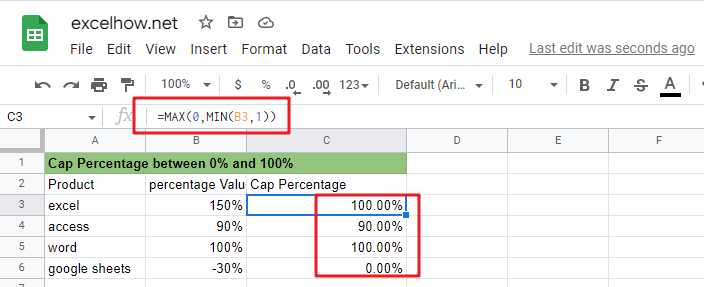If you are a newbie on Excel or google Sheets, you may be able to do this by manually. For tasks with small amounts of data, this method is possible, but for tasks with large amounts of data, this is not a wise method because it will take a lot of your time.

How to quickly cap a set of percentage values from 0% to 100%? You can now read the following section and we will talk about how to do this with formulas from Microsoft Excel or google sheets.

## Limiting percentages by MAX and MIN functions

You can use the MIN function in Google Sheets or Microsoft Excel Spreadsheet in combination with the MAX function to cap a given percentage value between 0% and 100%. Or you can do it with the IF nested functions.

Suppose we have a percentage value of 50%, which is greater than 0 and less than 100%, so it should return that percentage value; if the given percentage value is 120%, it is greater than 100%, so it should return 100%; if the given percentage value is -50%, it is less than 0%, so it should return 0%.

The general formula to achieve this task is as follows.

`=MAX(0,MIN(B3,1))`By applying this formula, you can always cap the percentage value between 0% and 100%.

### Formula explanation

Let’s see how this formula works.

#### =MIN(B3,1)

MIN function can be used to cap a given percentage value greater than 100%, returning 100%.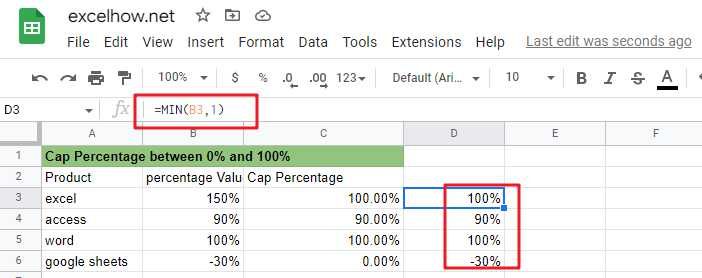#### =MAX(0,B3)

MAX function can be used to limit the given percentage value less than 0, return 0%.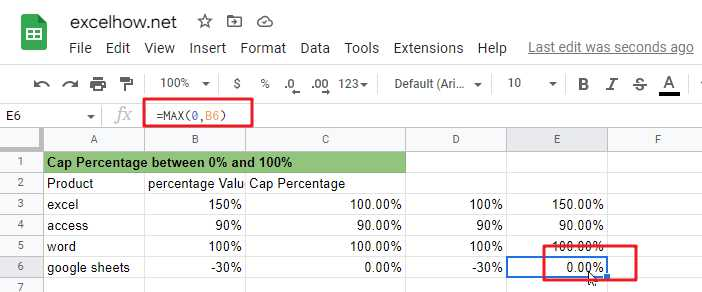## Limiting the percentage value by IF nested functions

You can also limit a given percentage value between 0% and 100% by using the IF nested function. The IF nested function is slightly more complex than the MAX/MIN nested function. There are three kinds of logic.

#### a) limit the value of the percentage does not exceed 100%

by the following IF formula can be used to limit the percentage value greater than 100%, so that the return value of 100%.

`=IF(B3>1,1,B3)`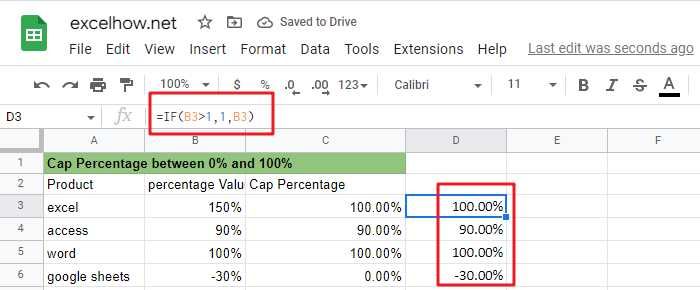#### b) Limit the percentage value not to be less than 0%

The following IF formula can be used to limit the percentage value less than 0, so that its return value is 0%

`=IF(B3<0,0,B3)`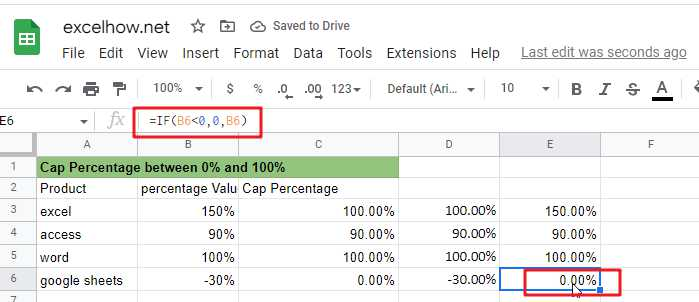#### c) Limit the percentage value that is greater than 0 and less than 100% and returns the original percentage value.

The above three selection logics can be satisfied simultaneously by the following nested IF formulas.

`=IF(B3>1,1,IF(B3<0,0,B3))`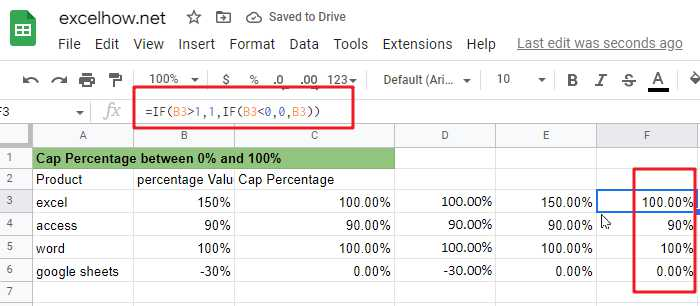From the screenshot above, you can see that the IF nested formula returns the same value as the MAX/MIN formula.

### Related Functions

The Google Sheets IF function perform a logical test to return one value if the condition is TRUE and return another value if the condition is FALSE. The IF function is a build-in function in Google Sheets and it is categorized as a Logical Function.The syntax of the IF function is as below:= IF (condition, [true_value],
The Google Sheets MIN function returns the smallest numeric value from the numbers that you provided. Or returns the smallest value in the array.The MIN function is a build-in function in Google Sheets and it is categorized as a Statistical Function.The syntax of the MIN function is as below:= MIN(num1,[num2,…numn])….
The Google Sheets MAX function returns the largest numeric value from the numbers that you provided. Or returns the largest value in the array.= MAX(num1,[num2,…numn])…

## Calculate Cap Percentages to Specific Value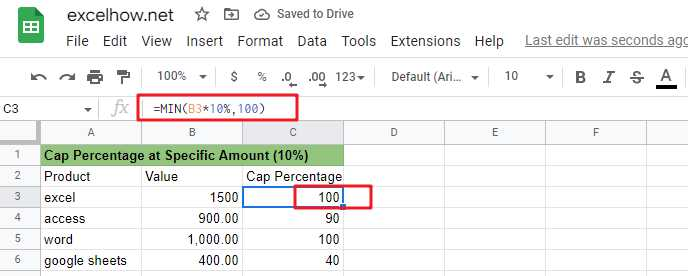If you are a newbie on Excel or google Sheets, you may be able to do this task manually. This method works for tasks with a small amount of data, but it is not a wise method for large amounts of data because it will consume a lot of your time.

How can you quickly calculate the cap percentage of a set of amount values? When you calculated the cap percentage of the amount, how do you limit that value to a given value? You can read the following section where we will explain how to do this with formulas in Microsoft Excel or google sheets.

## Calculate the cap percentage on a given amount

You can use the MIN function in Google Sheets or Microsoft Excel Spreadsheet to limit the cap percentage of a given amount to a specific value.

Suppose we have an amount value of 1500 and we need to calculate the upper limit of ten percent of that amount value and return 100 if the calculated cap value is greater than 100, or return that cap value if the calculated cap value is less than 100.

The general formula to complete this task is as follows.

`=MIN(B1*percentage,100)`

By executing this formula, you can always limit the cap percentage value of the amount value to 100.### Explanation of the formula

Let’s look at how this formula works：

Here are the three logics implemented in this formula.

• The MIN formula returns the cap percentage value when the calculated cap percentage value is less than 100.
• The MIN formula returns 100 when the calculated upper percentage value is greater than 100.
• The MIN formula returns 100 when the calculated upper percentage value is equal to 100.

From the above explanation, we can see that the MIN function can return the smallest value between the two values, but also can be used to achieve similar to the IF function can be used to select a specific value of the processing logic. Therefore, sometimes we can also use the MIN function instead of the IF function to complete our task.

### Related Functions

The Google Sheets IF function perform a logical test to return one value if the condition is TRUE and return another value if the condition is FALSE. The IF function is a build-in function in Google Sheets and it is categorized as a Logical Function.The syntax of the IF function is as below:= IF (condition, [true_value],
The Google Sheets MIN function returns the smallest numeric value from the numbers that you provided. Or returns the smallest value in the array.The MIN function is a build-in function in Google Sheets and it is categorized as a Statistical Function.The syntax of the MIN function is as below:= MIN(num1,[num2,…numn])….

## Google Sheets: Sort by Second or Third character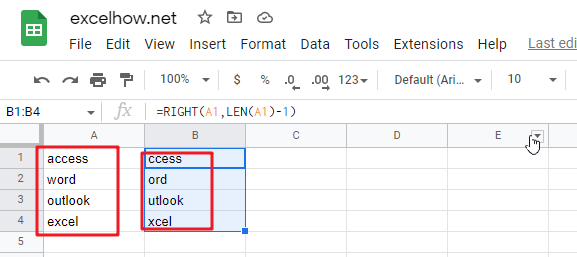This article will talk about how to sort by the second or third character in a cell’s string value in google sheets. You may want to sort the values of strings by the second or last character from the cells in google sheets, how can you do this task quickly? You can use the RIGHT function to create a google sheets formula to solve this problem. The following will show the detailed step-by-step instructions .

## Using the formula to rank by the second character

To sort the test string in the cell by its second character, you can use the RIGHT function and the combination of LEN function to create a new google sheets formula, you could refer to the general formula below.

`=RIGHT(Cell, LEN(Cell)-1)`

This formula will remove the first character of the text string in Cell, then you can use the sort feature to sort this column in google sheets, and then the original column will be sorted as well. Please do it as below.

Suppose you want to sort the A1:A4 range in column A by the second character.

1# Select cell B1 next to cell A1 that contains the data to be sorted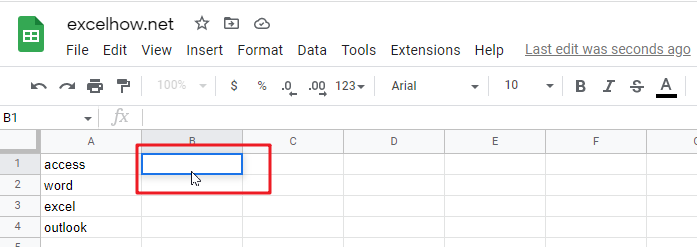2# Enter the following formula in the formula box in cell B1, and then press Enter.

`=right(a1,len(a1)-1)`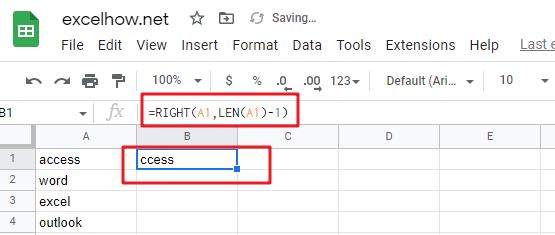3# Select cell B1, then drag the AutoFill handle down to cells B2:B4 to apply this formula. You will see that the first character will be removed.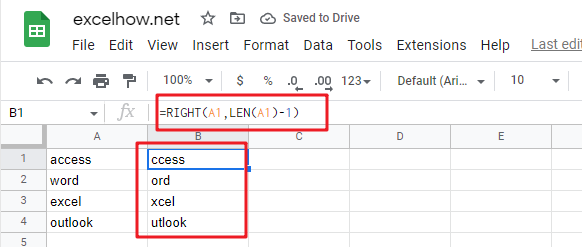4# Select cell B1:B4, click the “Data” menu, and then click the “Sort sheet by column B” submenu under the “Sort sheet” menu.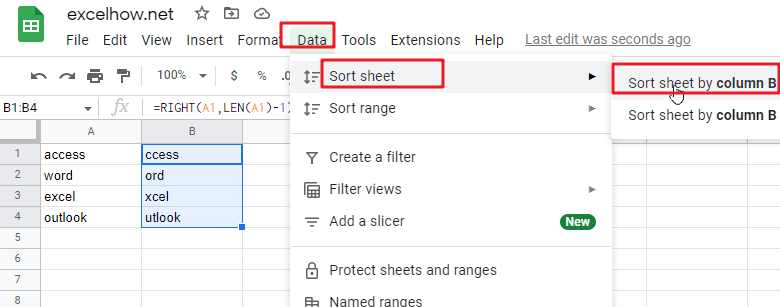5# You would see both column A and column B are sorted by the second character.6# You can now delete column B.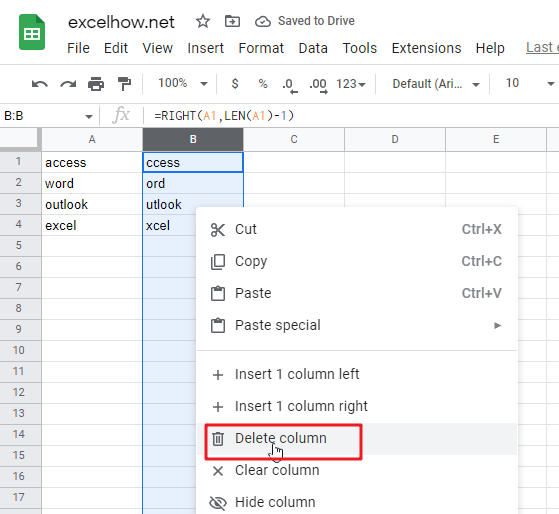If you want to sort by the third character, then you can use the following RIGHT formula, and then follow steps 3-7 to complete the sorting task.

`=RIGHT(Cell, LEN(Cell)-2)`

### Related Functions

The Google Sheets RIGHT function returns a substring (a specified number of the characters) from a text string, starting from the rightmost character.The syntax of the RIGHT function is as below:=RIGHT(text,[num_chars])…
The google sheetsLEN function returns the length of a text string (the number of characters in a text string).The syntax of the LEN function is as below:= LEN(text)…

## Sort Data by Last Character in google sheets

Sort Data by Last Character in google sheets

If you are an avid Google Sheets user, then you may have an urgent task to rank the text value by the last character from the cells; you can do this task manually very easily, which is also feasible, but only for sorting a small list of data. However, when there are a number of lists of data to be processed, it becomes a cumbersome and time-consuming task to sort by the last character by manually.

But don’t worry, because this article will guide you how to sort the data in a given cell range by the last character in google sheets.

## Sort Data by Last Character

Suppose you have a list of data in the range B1:B5 that contains text string values. You need to rank the cells based on the last character in the cell. You can easily rank the cell range by the first character by using the “Sort range” function in google sheets, but there is no function or command to sort the cell range by the last character directly in google sheets. So how can we achieve this task. You can use the helper column, and then use the RIGHT function to extract the last character of the string in the cell, and then you can sort the characters in the helper column. The following are the steps.

#1 Enter the following formula in cell C1.

`=RIGHT(A1,1)`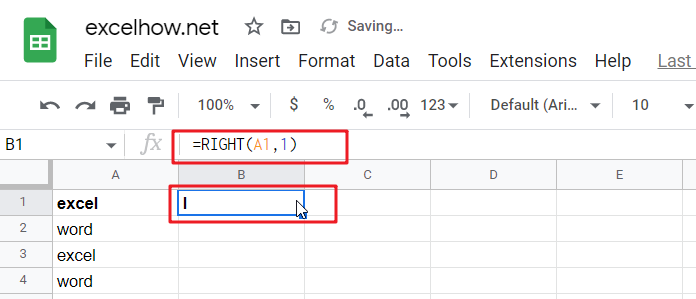#2 Press Enter on the keyboard, then drag the fill handle down to the other cells.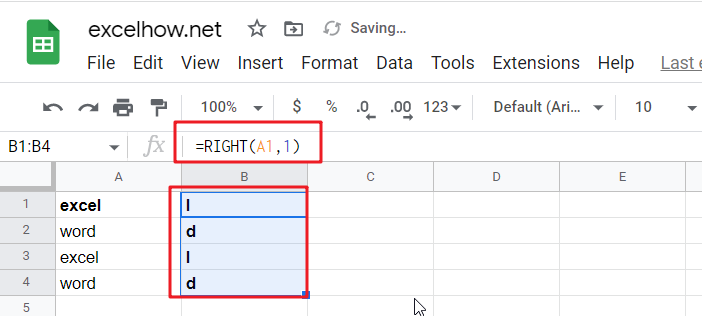#3 You need to continue to select the cells in the helper column, and then go to the “Data” tab, click “Sort sheet” menu and click the “Sort sheet by column B (A to Z)” submenu.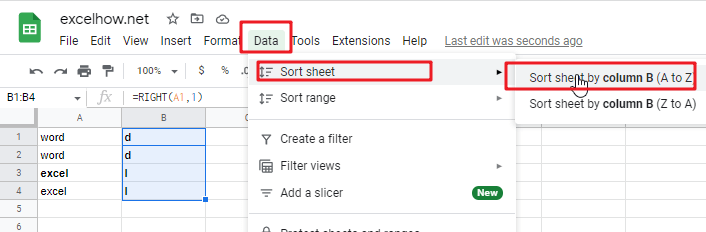#4 You should notice that the range of cells has been sorted by the last character. You can now delete the helper column.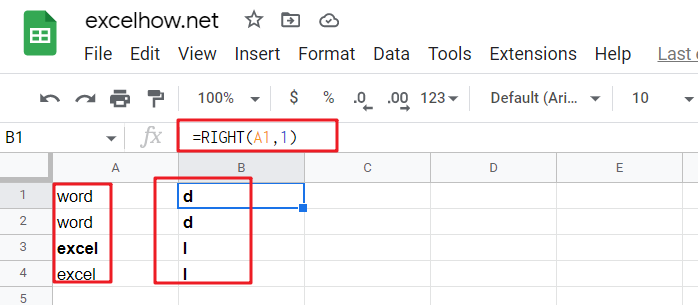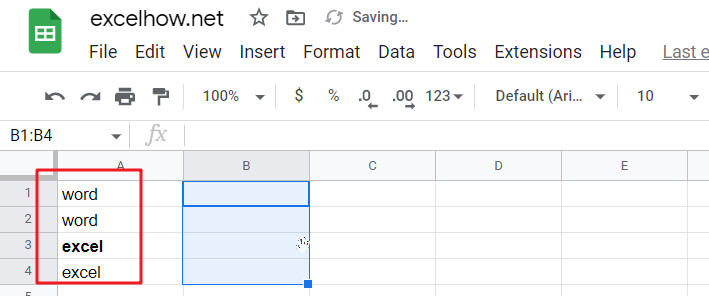### Related Functions

The Google Sheets RIGHT function returns a substring (a specified number of the characters) from a text string, starting from the rightmost character.The syntax of the RIGHT function is as below:=RIGHT(text,[num_chars])…

## Rank Data with Multiple Criteria in google sheets

If you are an enthusiastic Google Sheets user, then you may have an urgent task to rank a specified datelist based on given multiple criteria; you can easily perform this task manually, which is also feasible, but it only works for ranking a small amount of data. However, when there are multiple pieces of data to be processed, it becomes a cumbersome and time-consuming task to sort the list of data based on multiple conditions by manually.

But don’t worry, because this article will show you how to rank with multiple criteria in google sheets.

RANK Data with Multiple Criteria

If you want to rank the specified data by just one criteria, you can use the RANK function to easily rank the values of the range of cells in google sheets. Suppose you want to rank the data of a range of cells in a worksheet with multiple criteria. So how do you accomplish that task? You need to create a new complex formula by using the SUMPRODUCT function. For example, when you want to sort the list of data in the range of cells A1:B4 by using two criteria or conditions, simply use the following formula.

`=1+SUMPRODUCT((\$A\$1:\$A\$4=A1)*(\$B\$1:\$B\$4>B1))`

Next, you need to type this formula in the formula box in cell C1, then press Enter and drag the AutoFill handler to the other cells.

Let’s see the results.